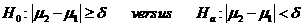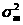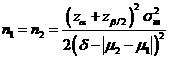# Sample Size Calculator: Two Crossover-Sample Means

Hypothesis: One-Sided EquivalenceData Input: (Help) (Example)

 Input Results α β Allowable difference n Population variance δ

Note:

 Variables Descriptions α One-sided significance level 1-β Power of the test Allowable difference Acceptable mean difference between sample two and sample one (µ2-µ1) Population variance Population varianceδ Equivalence limit n Sample size of each group

Help Aids Top

Application: Consider a 2×2 cross-over design contains two sequences (treatment orderings) and two time periods (occasions). One sequence receives treatment A followed by treatment B. The other sequence receives B and then A. This procedure is used to test the following hypotheses:Procedure:

1. Enter

a)      value of α, the probability of type I error

b)      value of β, the probability of type II error

c)      value of allowable difference

d)     value of population variance

e)      value of δ>0, the equivalence limit.

1. Click the button “Calculate” to obtain result sample size of each group n.

Formula:(*)

Notations:

α:               The probability of type I error (significance level) is the probability of rejecting the true null hypothesis.

β:               The probability of type II error (1 – power of the test) is the probability of failing to reject the false null hypothesis.

δ:               The largest change from the reference value (baseline) that is considered to be trivial.

μ2 – μ1:      The value of allowable difference is the true mean difference between a test drugs (μ2) and a placebo control or active control agent (μ1).

Examples

Example 1: Suppose a difference of 5% (i.e., δ=5%) in percent change of low density lipidproteins (LDLs) is considered of clinically meaningful difference. By using (*), assuming that the standard deviation is =0.2 (i.e., population variance is 0.04), the required sample size of each group to achieve an 80% power (β=0.2) at α=0.05 for correctly detecting such difference of μ2 – μ1=0 change obtained by normal approximation as n≈69.

Reference: Chow, Shao and Wang, Sample Size Calculations In Clinical Research, Taylor & Francis, NY. (2003) Pages 66-67.